# Vaex introduction in 11 minutes¶

Because vaex goes up to 11

If you want to try out this notebook with a live Python kernel, use mybinder:## DataFrame¶

Central to Vaex is the DataFrame (similar, but more efficient than a Pandas DataFrame), and we often use the variable df to represent it. A DataFrame is an efficient representation for a large tabular dataset, and has:

• A number of columns, say x, y and z, which are:
• Backed by a Numpy array;
• Wrapped by an expression system e.g. df.x, df['x'] or df.col.x is an Expression;
• Columns/expression can perform lazy computations, e.g. df.x * np.sin(df.y) does nothing, until the result is needed.
• A set of virtual columns, columns that are backed by a (lazy) computation, e.g. df['r'] = df.x/df.y
• A set of selections, that can be used to explore the dataset, e.g. df.select(df.x < 0)
• Filtered DataFrames, that does not copy the data, df_negative = df[df.x < 0]

:

import vaex
df = vaex.example()
df  # Since this is the last statement in a cell, it will print the DataFrame in a nice HTML format.

:

# id x y z vx vy vz E L Lz FeH
0 0 1.2318683862686157 -0.39692866802215576-0.598057746887207 301.1552734375 174.05947875976562 27.42754554748535 -149431.40625 407.38897705078125333.9555358886719 -1.0053852796554565
1 23 -0.163700610399246223.654221296310425 -0.25490644574165344-195.00022888183594170.47216796875 142.5302276611328 -124247.953125890.2411499023438 684.6676025390625 -1.7086670398712158
2 32 -2.120255947113037 3.326052665710449 1.7078403234481812 -48.63423156738281 171.6472930908203 -2.079437255859375 -138500.546875372.2410888671875 -202.17617797851562-1.8336141109466553
3 8 4.7155890464782715 4.5852508544921875 2.2515437602996826 -232.42083740234375-294.850830078125 62.85865020751953 -60037.03906251297.63037109375 -324.6875 -1.4786882400512695
4 16 7.21718692779541 11.99471664428711 -1.064562201499939 -1.6891745328903198181.329345703125 -11.333610534667969-83206.84375 1332.79895019531251328.948974609375 -1.8570483922958374
... ... ... ... ... ... ... ... ... ... ... ...
329,99521 1.9938701391220093 0.789276123046875 0.22205990552902222 -216.9299011230468816.124420166015625 -211.244384765625 -146457.4375 457.72247314453125203.36758422851562 -1.7451677322387695
329,99625 3.7180912494659424 0.721337616443634 1.6415337324142456 -185.92160034179688-117.25082397460938-105.4986572265625 -126627.109375335.0025634765625 -301.8370056152344 -0.9822322130203247
329,99714 0.3688507676124573 13.029608726501465 -3.633934736251831 -53.677146911621094-145.15771484375 76.70909881591797 -84912.2578125817.1375732421875 645.8507080078125 -1.7645612955093384
329,99818 -0.112592644989490511.4529125690460205 2.168952703475952 179.30865478515625 205.79710388183594 -68.75872802734375 -133498.46875 724.000244140625 -283.6910400390625 -1.8808952569961548
329,9994 20.796220779418945 -3.331387758255005 12.18841552734375 42.69000244140625 69.20479583740234 29.54275131225586 -65519.328125 1843.07470703125 1581.4151611328125 -1.1231083869934082

### Columns¶

The above preview shows that this dataset contains $$> 300,000$$ rows, and columns named x ,y, z (positions), vx, vy, vz (velocities), E (energy), L (angular momentum), and an id (subgroup of samples). When we print out a columns, we can see that it is not a Numpy array, but an Expression.

:

df.x  # df.col.x or df['x'] are equivalent, but df.x may be preferred because it is more tab completion friendly or programming friendly respectively

:

Expression = x
Length: 330,000 dtype: float32 (column)
---------------------------------------
0    1.23187
1  -0.163701
2   -2.12026
3    4.71559
4    7.21719
...
329995    1.99387
329996    3.71809
329997   0.368851
329998  -0.112593
329999    20.7962


One can use the .values method to get an in-memory representation of an expression. The same method can be applied to a DataFrame as well.

:

df.x.values

:

array([ 1.2318684 , -0.16370061, -2.120256  , ...,  0.36885077,
-0.11259264, 20.79622   ], dtype=float32)


Most Numpy functions (ufuncs) can be performed on expressions, and will not result in a direct result, but in a new expression.

:

import numpy as np
np.sqrt(df.x**2 + df.y**2 + df.z**2)

:

Expression = sqrt((((x ** 2) + (y ** 2)) + (z ** 2)))
Length: 330,000 dtype: float32 (expression)
-------------------------------------------
0  1.42574
1  3.66676
2  4.29824
3  6.95203
4   14.039
...
329995  2.15587
329996  4.12785
329997  13.5319
329998  2.61304
329999  24.3339


### Virtual columns¶

Sometimes it is convenient to store an expression as a column. We call this a virtual column since it does not take up any memory, and is computed on the fly when needed. A virtual column is treated just as a normal column.

:

df['r'] = np.sqrt(df.x**2 + df.y**2 + df.z**2)
df[['x', 'y', 'z', 'r']]

:

# x y z r
0 1.2318683862686157 -0.39692866802215576-0.598057746887207 1.425736665725708
1 -0.163700610399246223.654221296310425 -0.254906445741653443.666757345199585
2 -2.120255947113037 3.326052665710449 1.7078403234481812 4.298235893249512
3 4.7155890464782715 4.5852508544921875 2.2515437602996826 6.952032566070557
4 7.21718692779541 11.99471664428711 -1.064562201499939 14.03902816772461
... ... ... ... ...
329,9951.9938701391220093 0.789276123046875 0.22205990552902222 2.155872344970703
329,9963.7180912494659424 0.721337616443634 1.6415337324142456 4.127851963043213
329,9970.3688507676124573 13.029608726501465 -3.633934736251831 13.531896591186523
329,998-0.112592644989490511.4529125690460205 2.168952703475952 2.613041877746582
329,99920.796220779418945 -3.331387758255005 12.18841552734375 24.333894729614258

### Selections and filtering¶

Vaex can be efficient when exploring subsets of the data, for instance to remove outliers or to inspect only a part of the data. Instead of making copies, Vaex internally keeps track which rows are selected.

:

df.select(df.x < 0)
df.evaluate(df.x, selection=True)

:

array([-0.16370061, -2.120256  , -7.7843747 , ..., -8.126636  ,
-3.9477386 , -0.11259264], dtype=float32)


Selections are useful when you frequently modify the portion of the data you want to visualize, or when you want to efficiently compute statistics on several portions of the data effectively.

Alternatively, you can also create filtered datasets. This is similar to using Pandas, except that Vaex does not copy the data.

:

df_negative = df[df.x < 0]
df_negative[['x', 'y', 'z', 'r']]

:

# x y z r
0 -0.163700610399246223.654221296310425 -0.254906445741653443.666757345199585
1 -2.120255947113037 3.326052665710449 1.7078403234481812 4.298235893249512
2 -7.784374713897705 5.989774703979492 -0.682695209980011 9.845809936523438
3 -3.5571861267089844 5.413629055023193 0.09171556681394577 6.478376865386963
4 -20.813940048217773 -3.294677495956421 13.486607551574707 25.019264221191406
... ... ... ... ...
166,274-2.5926425457000732 -2.871671676635742 -0.180483341217041023.8730955123901367
166,275-0.7566012144088745 2.9830434322357178 -6.940553188323975 7.592250823974609
166,276-8.126635551452637 1.1619765758514404 -1.6459038257598877 8.372657775878906
166,277-3.9477386474609375 -3.0684902667999268-1.5822702646255493 5.244411468505859
166,278-0.112592644989490511.4529125690460205 2.168952703475952 2.613041877746582

## Statistics on N-d grids¶

A core feature of Vaex is the extremely efficient calculation of statistics on N-dimensional grids. The is rather useful for making visualisations of large datasets.

:

df.count(), df.mean(df.x), df.mean(df.x, selection=True)

:

(array(330000), array(-0.0632868), array(-5.18457762))


Similar to SQL’s groupby, Vaex uses the binby concept, which tells Vaex that a statistic should be calculated on a regular grid (for performance reasons)

:

counts_x = df.count(binby=df.x, limits=[-10, 10], shape=64)
counts_x

:

array([1374, 1350, 1459, 1618, 1706, 1762, 1852, 2007, 2240, 2340, 2610,
2840, 3126, 3337, 3570, 3812, 4216, 4434, 4730, 4975, 5332, 5800,
6162, 6540, 6805, 7261, 7478, 7642, 7839, 8336, 8736, 8279, 8269,
8824, 8217, 7978, 7541, 7383, 7116, 6836, 6447, 6220, 5864, 5408,
4881, 4681, 4337, 4015, 3799, 3531, 3320, 3040, 2866, 2629, 2488,
2244, 1981, 1905, 1734, 1540, 1437, 1378, 1233, 1186])


This results in a Numpy array with the number counts in 64 bins distributed between x = -10, and x = 10. We can quickly visualize this using Matplotlib.

:

import matplotlib.pylab as plt
plt.plot(np.linspace(-10, 10, 64), counts_x)
plt.show()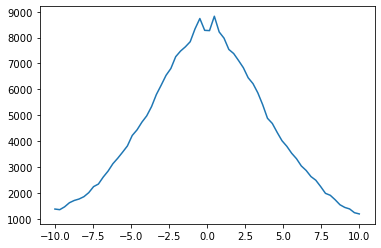We can do the same in 2D as well (this can be generalized to N-D actually!), and display it with Matplotlib.

:

xycounts = df.count(binby=[df.x, df.y], limits=[[-10, 10], [-10, 20]], shape=(64, 128))
xycounts

:

array([[ 5,  2,  3, ...,  3,  3,  0],
[ 8,  4,  2, ...,  5,  3,  2],
[ 5, 11,  7, ...,  3,  3,  1],
...,
[ 4,  8,  5, ...,  2,  0,  2],
[10,  6,  7, ...,  1,  1,  2],
[ 6,  7,  9, ...,  2,  2,  2]])

:

plt.imshow(xycounts.T, origin='lower', extent=[-10, 10, -10, 20])
plt.show()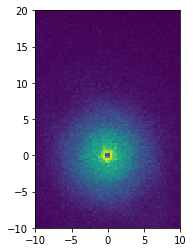:

v = np.sqrt(df.vx**2 + df.vy**2 + df.vz**2)
xy_mean_v = df.mean(v, binby=[df.x, df.y], limits=[[-10, 10], [-10, 20]], shape=(64, 128))
xy_mean_v

:

array([[156.15283203, 226.0004425 , 206.95940653, ...,  90.0340627 ,
152.08784485,          nan],
[203.81366634, 133.01436043, 146.95962524, ..., 137.54756927,
98.68717448, 141.06020737],
[150.59178772, 188.38820371, 137.46753802, ..., 155.96900177,
148.91660563, 138.48191833],
...,
[168.93819809, 187.75943136, 137.318647  , ..., 144.83927917,
nan, 107.7273407 ],
[154.80492783, 140.55182203, 180.30700166, ..., 184.01670837,
95.10913086, 131.18122864],
[166.06868235, 150.54079764, 125.84606828, ..., 130.56007385,
121.04217911, 113.34659195]])

:

plt.imshow(xy_mean_v.T, origin='lower', extent=[-10, 10, -10, 20])
plt.show()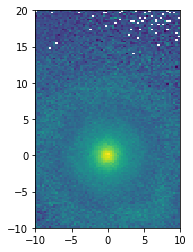Other statistics can be computed, such as:

Or see the full list at the API docs.

Before continuing with this tutorial, you may want to read in your own data. Ultimately, a Vaex DataFrame just wraps a set of Numpy arrays. If you can access your data as a set of Numpy arrays, you can easily construct a DataFrame using from_arrays.

:

import vaex
import numpy as np
x = np.arange(5)
y = x**2
df = vaex.from_arrays(x=x, y=y)
df

:

# x y
0 0 0
1 1 1
2 2 4
3 3 9
4 4 16

Other quick ways to get your data in are:

Exporting, or converting a DataFrame to a different datastructure is also quite easy:

Nowadays, it is common to put data, especially larger dataset, on the cloud. Vaex can read data straight from S3, in a lazy manner, meaning that only that data that is needed will be downloaded, and cached on disk.

:

# Read in the NYC Taxi dataset straight from S3
nyctaxi = vaex.open('s3://vaex/taxi/yellow_taxi_2009_2015_f32.hdf5?anon=true')

:

# vendor_id pickup_datetime dropoff_datetime passenger_countpayment_type trip_distance pickup_longitude pickup_latitude rate_code store_and_fwd_flag dropoff_longitude dropoff_latitude fare_amount surcharge mta_tax tip_amount tolls_amount total_amount
0VTS 2009-01-04 02:52:00.0000000002009-01-04 03:02:00.000000000 1CASH 2.63 -73.992 40.7216 nan nan -73.9938 40.6959 8.9 0.5 nan 0 0 9.4
1VTS 2009-01-04 03:31:00.0000000002009-01-04 03:38:00.000000000 3Credit 4.55 -73.9821 40.7363 nan nan -73.9558 40.768 12.1 0.5 nan 2 0 14.6
2VTS 2009-01-03 15:43:00.0000000002009-01-03 15:57:00.000000000 5Credit 10.35 -74.0026 40.7397 nan nan -73.87 40.7702 23.7 0 nan 4.74 0 28.44
3DDS 2009-01-01 20:52:58.0000000002009-01-01 21:14:00.000000000 1CREDIT 5 -73.9743 40.791 nan nan -73.9966 40.7318 14.9 0.5 nan 3.05 0 18.45
4DDS 2009-01-24 16:18:23.0000000002009-01-24 16:24:56.000000000 1CASH 0.4 -74.0016 40.7194 nan nan -74.0084 40.7203 3.7 0 nan 0 0 3.7

## Plotting¶

### 1-D and 2-D¶

Most visualizations are done in 1 or 2 dimensions, and Vaex nicely wraps Matplotlib to satisfy a variety of frequent use cases.

:

import vaex
import numpy as np
df = vaex.example()


The simplest visualization is a 1-D plot using DataFrame.plot1d. When only given one argument, it will show a histogram showing 99.7% of the data.

:

df.plot1d(df.x, limits='99.7%');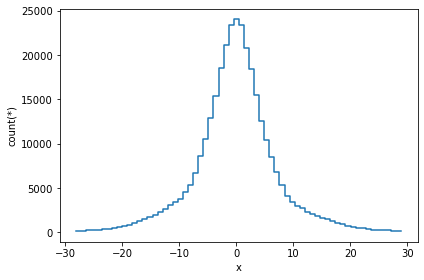A slighly more complication visualization, is to plot not the counts, but a different statistic for that bin. In most cases, passing the what='<statistic>(<expression>) argument will do, where <statistic> is any of the statistics mentioned in the list above, or in the API docs.

:

df.plot1d(df.x, what='mean(E)', limits='99.7%');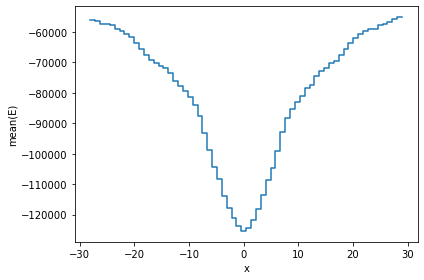An equivalent method is to use the vaex.stat.<statistic> functions, e.g. vaex.stat.mean.

:

df.plot1d(df.x, what=vaex.stat.mean(df.E), limits='99.7%');The vaex.stat.<statistic> objects are very similar to Vaex expressions, in that they represent an underlying calculation. Typical arithmetic and Numpy functions can be applied to these calulations. However, these objects compute a single statistic, and do not return a column or expression.

:

np.log(vaex.stat.mean(df.x)/vaex.stat.std(df.x))

:

log((mean(x) / std(x)))


These statistical objects can be passed to the what argument. The advantage being that the data will only have to be passed over once.

:

df.plot1d(df.x, what=np.clip(np.log(-vaex.stat.mean(df.E)), 11, 11.4), limits='99.7%');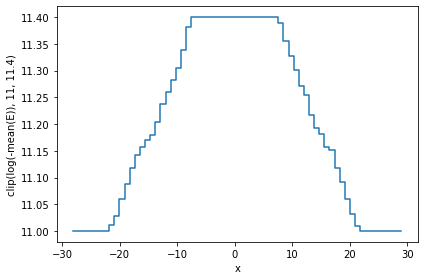A similar result can be obtained by calculating the statistic ourselves, and passing it to plot1d’s grid argument. Care has to be taken that the limits used for calculating the statistics and the plot are the same, otherwise the x axis may not correspond to the real data.

:

limits = [-30, 30]
shape  = 64
meanE  = df.mean(df.E, binby=df.x, limits=limits, shape=shape)
grid   = np.clip(np.log(-meanE), 11, 11.4)
df.plot1d(df.x, grid=grid, limits=limits, ylabel='clipped E');The same applies for 2-D plotting.

:

df.plot(df.x, df.y, what=vaex.stat.mean(df.E)**2, limits='99.7%');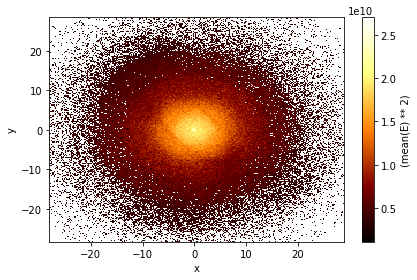### Selections for plotting¶

While filtering is useful for narrowing down the contents of a DataFrame (e.g. df_negative = df[df.x < 0]) there are a few downsides to this. First, a practical issue is that when you filter 4 different ways, you will need to have 4 different DataFrames polluting your namespace. More importantly, when Vaex executes a bunch of statistical computations, it will do that per DataFrame, meaning that 4 passes over the data will be made, and even though all 4 of those DataFrames point to the same underlying data.

If instead we have 4 (named) selections in our DataFrame, we can calculate statistics in one single pass over the data, which can be significantly faster especially in the cases when your dataset is larger than your memory.

In the plot below we show three selection, which by default are blended together, requiring just one pass over the data.

:

df.plot(df.x, df.y, what=np.log(vaex.stat.count()+1), limits='99.7%',
selection=[None, df.x < df.y, df.x < -10]);

/Users/jovan/PyLibrary/vaex/packages/vaex-core/vaex/image.py:113: FutureWarning: Using a non-tuple sequence for multidimensional indexing is deprecated; use arr[tuple(seq)] instead of arr[seq]. In the future this will be interpreted as an array index, arr[np.array(seq)], which will result either in an error or a different result.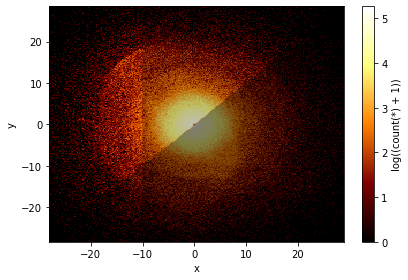Lets say we would like to see two plots next to eachother. To achieve this we can pass a list of expression pairs.

:

df.plot([["x", "y"], ["x", "z"]], limits='99.7%',
title="Face on and edge on", figsize=(10,4));

/Users/jovan/PyLibrary/vaex/packages/vaex-core/vaex/viz/mpl.py:779: MatplotlibDeprecationWarning: Adding an axes using the same arguments as a previous axes currently reuses the earlier instance.  In a future version, a new instance will always be created and returned.  Meanwhile, this warning can be suppressed, and the future behavior ensured, by passing a unique label to each axes instance.
ax = pylab.subplot(gs[row_offset + row * row_scale:row_offset + (row + 1) * row_scale, column * column_scale:(column + 1) * column_scale])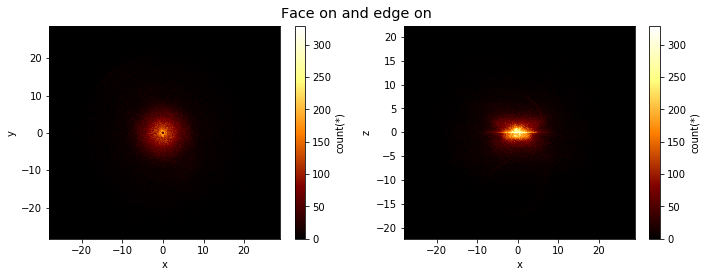By default, if you have multiple plots, they are shown as columns, multiple selections are overplotted, and multiple ‘whats’ (statistics) are shown as rows.

:

df.plot([["x", "y"], ["x", "z"]],
limits='99.7%',
what=[np.log(vaex.stat.count()+1), vaex.stat.mean(df.E)],
selection=[None, df.x < df.y],
title="Face on and edge on", figsize=(10,10));Note that the selection has no effect in the bottom rows.

However, this behaviour can be changed using the visual argument.

:

df.plot([["x", "y"], ["x", "z"]],
limits='99.7%',
what=vaex.stat.mean(df.E),
selection=[None, df.Lz < 0],
visual=dict(column='selection'),
title="Face on and edge on", figsize=(10,10));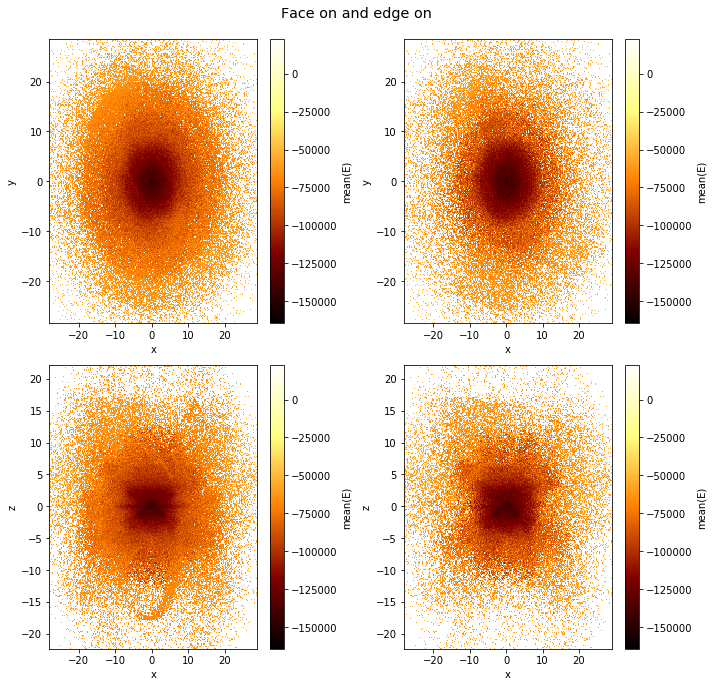### Slices in a 3rd dimension¶

If a 3rd axis (z) is given, you can ‘slice’ through the data, displaying the z slices as rows. Note that here the rows are wrapped, which can be changed using the wrap_columns argument.

:

df.plot("Lz", "E",
limits='99.7%',
z="FeH:-2.5,-1,8", show=True, visual=dict(row="z"),
figsize=(12,8), f="log", wrap_columns=3);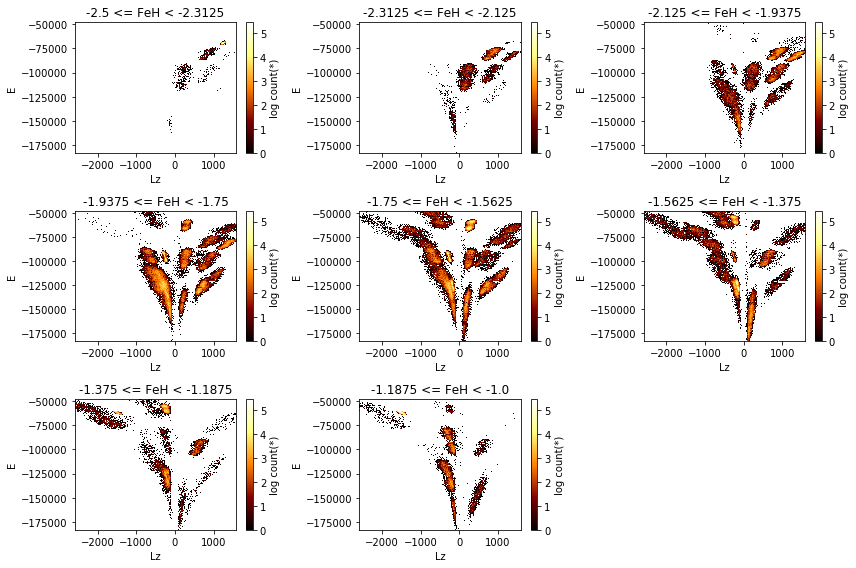### Visualization of smaller datasets¶

Although Vaex focuses on large datasets, sometimes you end up with a fraction of the data (e.g. due to a selection) and you want to make a scatter plot. You can do so with the following approach:

:

import vaex
df = vaex.example()

:

import matplotlib.pylab as plt
x = df.evaluate("x", selection=df.Lz < -2500)
y = df.evaluate("y", selection=df.Lz < -2500)
plt.scatter(x, y, c="red", alpha=0.5, s=4);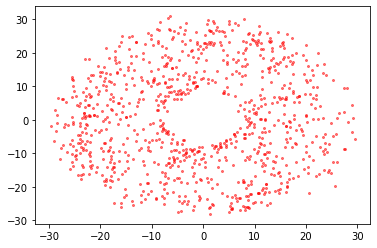:

df.scatter(df.x, df.y, selection=df.Lz < -2500, c="red", alpha=0.5, s=4)
df.scatter(df.x, df.y, selection=df.Lz > 1500, c="green", alpha=0.5, s=4);### In control¶

While Vaex provides a wrapper for Matplotlib, there are situations where you want to use the DataFrame.plot method, but want to be in control of the plot. Vaex simply uses the current figure and axes objects, so that it is easy to do.

:

fig, (ax1, ax2) = plt.subplots(1, 2, figsize=(14,7))
plt.sca(ax1)
selection = df.Lz < -2500
x = df[selection].x.evaluate()#selection=selection)
y = df[selection].y.evaluate()#selection=selection)
df.plot(df.x, df.y)
plt.scatter(x, y)
plt.xlabel('my own label $\gamma$')
plt.xlim(-20, 20)
plt.ylim(-20, 20)

plt.sca(ax2)
df.plot1d(df.x, label='counts', n=True)
x = np.linspace(-30, 30, 100)
std = df.std(df.x.expression)
y = np.exp(-(x**2/std**2/2)) / np.sqrt(2*np.pi) / std
plt.plot(x, y, label='gaussian fit')
plt.legend()
plt.show()### Healpix (Plotting)¶

Healpix plotting is supported via the healpy package. Vaex does not need special support for healpix, only for plotting, but some helper functions are introduced to make working with healpix easier.

In the following example we will use the TGAS astronomy dataset.

To understand healpix better, we will start from the beginning. If we want to make a density sky plot, we would like to pass healpy a 1D Numpy array where each value represents the density at a location of the sphere, where the location is determined by the array size (the healpix level) and the offset (the location). The TGAS (and Gaia) data includes the healpix index encoded in the source_id. By diving the source_id by 34359738368 you get a healpix index level 12, and diving it further will take you to lower levels.

:

import vaex
import healpy as hp
tgas = vaex.datasets.tgas.fetch()


We will start showing how you could manually do statistics on healpix bins using vaex.count. We will do a really course healpix scheme (level 2).

:

level = 2
factor = 34359738368 * (4**(12-level))
nmax = hp.nside2npix(2**level)
epsilon = 1e-16
counts = tgas.count(binby=tgas.source_id/factor, limits=[-epsilon, nmax-epsilon], shape=nmax)
counts

:

array([ 4021,  6171,  5318,  7114,  5755, 13420, 12711, 10193,  7782,
14187, 12578, 22038, 17313, 13064, 17298, 11887,  3859,  3488,
9036,  5533,  4007,  3899,  4884,  5664, 10741,  7678, 12092,
10182,  6652,  6793, 10117,  9614,  3727,  5849,  4028,  5505,
8462, 10059,  6581,  8282,  4757,  5116,  4578,  5452,  6023,
8340,  6440,  8623,  7308,  6197, 21271, 23176, 12975, 17138,
26783, 30575, 31931, 29697, 17986, 16987, 19802, 15632, 14273,
10594,  4807,  4551,  4028,  4357,  4067,  4206,  3505,  4137,
3311,  3582,  3586,  4218,  4529,  4360,  6767,  7579, 14462,
24291, 10638, 11250, 29619,  9678, 23322, 18205,  7625,  9891,
5423,  5808, 14438, 17251,  7833, 15226,  7123,  3708,  6135,
4110,  3587,  3222,  3074,  3941,  3846,  3402,  3564,  3425,
4125,  4026,  3689,  4084, 16617, 13577,  6911,  4837, 13553,
10074,  9534, 20824,  4976,  6707,  5396,  8366, 13494, 19766,
11012, 16130,  8521,  8245,  6871,  5977,  8789, 10016,  6517,
8019,  6122,  5465,  5414,  4934,  5788,  6139,  4310,  4144,
11437, 30731, 13741, 27285, 40227, 16320, 23039, 10812, 14686,
27690, 15155, 32701, 18780,  5895, 23348,  6081, 17050, 28498,
35232, 26223, 22341, 15867, 17688,  8580, 24895, 13027, 11223,
7880,  8386,  6988,  5815,  4717,  9088,  8283, 12059,  9161,
6952,  4914,  6652,  4666, 12014, 10703, 16518, 10270,  6724,
4553,  9282,  4981])


And using healpy’s mollview we can visualize this.

:

hp.mollview(counts, nest=True)To simplify life, Vaex includes DataFrame.healpix_count to take care of this.

:

counts = tgas.healpix_count(healpix_level=6)
hp.mollview(counts, nest=True)Or even simpler, use DataFrame.healpix_plot

:

tgas.healpix_plot(f="log1p", healpix_level=6, figsize=(10,8),
healpix_output="ecliptic")## xarray suppport¶

The df.count method can also return an xarray data array instead of a numpy array. This is easily done via the array_type keyword. Building on top of numpy, xarray adds dimension labels, coordinates and attributes, that makes working with multi-dimensional arrays more convenient.

:

xarr = df.count(binby=[df.x, df.y], limits=[-10, 10], shape=64, array_type='xarray')
xarr

:

xarray.DataArray
• x: 64
• y: 64
• 6 3 7 9 10 13 6 13 17 7 12 15 7 14 ... 11 8 10 6 7 5 17 9 10 10 6 5 7
array([[ 6,  3,  7, ..., 15, 10, 11],
[10,  3,  7, ..., 10, 13, 11],
[ 5, 15,  5, ..., 12, 18, 12],
...,
[ 7,  8, 10, ...,  6,  7,  7],
[12, 10, 17, ..., 11,  8,  2],
[ 7, 10, 13, ...,  6,  5,  7]])
• x
(x)
float64
-9.844 -9.531 ... 9.531 9.844
array([-9.84375, -9.53125, -9.21875, -8.90625, -8.59375, -8.28125, -7.96875,
-7.65625, -7.34375, -7.03125, -6.71875, -6.40625, -6.09375, -5.78125,
-5.46875, -5.15625, -4.84375, -4.53125, -4.21875, -3.90625, -3.59375,
-3.28125, -2.96875, -2.65625, -2.34375, -2.03125, -1.71875, -1.40625,
-1.09375, -0.78125, -0.46875, -0.15625,  0.15625,  0.46875,  0.78125,
1.09375,  1.40625,  1.71875,  2.03125,  2.34375,  2.65625,  2.96875,
3.28125,  3.59375,  3.90625,  4.21875,  4.53125,  4.84375,  5.15625,
5.46875,  5.78125,  6.09375,  6.40625,  6.71875,  7.03125,  7.34375,
7.65625,  7.96875,  8.28125,  8.59375,  8.90625,  9.21875,  9.53125,
9.84375])
• y
(y)
float64
-9.844 -9.531 ... 9.531 9.844
array([-9.84375, -9.53125, -9.21875, -8.90625, -8.59375, -8.28125, -7.96875,
-7.65625, -7.34375, -7.03125, -6.71875, -6.40625, -6.09375, -5.78125,
-5.46875, -5.15625, -4.84375, -4.53125, -4.21875, -3.90625, -3.59375,
-3.28125, -2.96875, -2.65625, -2.34375, -2.03125, -1.71875, -1.40625,
-1.09375, -0.78125, -0.46875, -0.15625,  0.15625,  0.46875,  0.78125,
1.09375,  1.40625,  1.71875,  2.03125,  2.34375,  2.65625,  2.96875,
3.28125,  3.59375,  3.90625,  4.21875,  4.53125,  4.84375,  5.15625,
5.46875,  5.78125,  6.09375,  6.40625,  6.71875,  7.03125,  7.34375,
7.65625,  7.96875,  8.28125,  8.59375,  8.90625,  9.21875,  9.53125,
9.84375])

In addition, xarray also has a plotting method that can be quite convenient. Since the xarray object has information about the labels of each dimension, the plot axis will be automatially labeled.

:

xarr.plot();Having xarray as output helps us to explore the contents of our data faster. In the following example we show how easy it is to plot the 2D distribution of the positions of the samples (x, y), per id group.

Notice how xarray automatically adds the appropriate titles and axis labels to the figure.

:

df.categorize('id', inplace=True)  # treat the id as a categorical column - automatically adjusts limits and shape
xarr = df.count(binby=['x', 'y', 'id'], limits='95%', array_type='xarray')
np.log1p(xarr).plot(col='id', col_wrap=7);## Interactive widgets¶

Note: The interactive widgets require a running Python kernel, if you are viewing this documentation online you can get a feeling for what the widgets can do, but computation will not be possible!

Using the vaex-jupyter package, we get access to interactive widgets (go see the Vaex Jupyter tutorial for a more in depth tutorial)

:

import vaex
import vaex.jupyter
import numpy as np
import pylab as plt
df = vaex.example()


The simplest way to get a more interactive visualization (or even print out statistics) is to use the vaex.jupyter.interactive_selection decorator, which will execute the decorated function each time the selection is changed.

:

df.select(df.x > 0)
@vaex.jupyter.interactive_selection(df)
def plot(*args, **kwargs):
print("Mean x for the selection is:", df.mean(df.x, selection=True))
df.plot(df.x, df.y, what=np.log(vaex.stat.count()+1), selection=[None, True], limits='99.7%')
plt.show()


After changing the selection programmatically, the visualization will update, as well as the print output.

:

df.select(df.x > df.y)


However, to get truly interactive visualization, we need to use widgets, such as the bqplot library. Again, if we make a selection here, the above visualization will also update, so lets select a square region.

See more interactive widgets in the Vaex Jupyter tutorial

## Joining¶

Joining in Vaex is similar to Pandas, except the data will no be copied. Internally an index array is kept for each row on the left DataFrame, pointing to the right DataFrame, requiring about 8GB for a billion row $$10^9$$ dataset. Lets start with 2 small DataFrames, df1 and df2:

:

a = np.array(['a', 'b', 'c'])
x = np.arange(1,4)
df1 = vaex.from_arrays(a=a, x=x)
df1

:

# a x
0a 1
1b 2
2c 3
:

b = np.array(['a', 'b', 'd'])
y = x**2
df2 = vaex.from_arrays(b=b, y=y)
df2

:

# b y
0a 1
1b 4
2d 9

The default join, is a ‘left’ join, where all rows for the left DataFrame (df1) are kept, and matching rows of the right DataFrame (df2) are added. We see that for the columns b and y, some values are missing, as expected.

:

df1.join(df2, left_on='a', right_on='b')

:

# a xb y
0a 1a 1
1b 2b 4
2c 3-- --

A ‘right’ join, is basically the same, but now the roles of the left and right label swapped, so now we have some values from columns x and a missing.

:

df1.join(df2, left_on='a', right_on='b', how='right')

:

# b ya x
0a 1a 1
1b 4b 2
2d 9-- --

We can also do ‘inner’ join, in which the output DataFrame has only the rows common between df1 and df2.

:

df1.join(df2, left_on='a', right_on='b', how='inner')

:

# a xb y
0a 1a 1
1b 2b 4

Other joins (e.g. outer) are currently not supported. Feel free to open an issue on GitHub for this.

## Group-by¶

With Vaex one can also do fast group-by aggregations. The output is Vaex DataFrame. Let us see few examples.

:

import vaex
animal = ['dog', 'dog', 'cat', 'guinea pig', 'guinea pig', 'dog']
age = [2, 1, 5, 1, 3, 7]
cuteness = [9, 10, 5, 8, 4, 8]
df_pets = vaex.from_arrays(animal=animal, age=age, cuteness=cuteness)
df_pets

:

# animal age cuteness
0dog 2 9
1dog 1 10
2cat 5 5
3guinea pig 1 8
4guinea pig 3 4
5dog 7 8

The syntax for doing group-by operations is virtually identical to that of Pandas. Note that when multiple aggregations are passed to a single column or expression, the output colums are appropriately named.

:

df_pets.groupby(by='animal').agg({'age': 'mean',
'cuteness': ['mean', 'std']})

:

# animal age cuteness_mean cuteness_std
0dog 3.33333 9 0.816497
1cat 5 5 0
2guinea pig2 6 2

Vaex supports a number of aggregation functions:

In addition, we can specify the aggregation operations inside the groupby-method. Also we can name the resulting aggregate columns as we wish.

:

df_pets.groupby(by='animal',
agg={'mean_age': vaex.agg.mean('age'),
'cuteness_unique_values': vaex.agg.nunique('cuteness'),
'cuteness_unique_min': vaex.agg.min('cuteness')})

:

# animal mean_age cuteness_unique_values cuteness_unique_min
0dog 3.33333 3 8
1cat 5 1 5
2guinea pig 2 2 4

A powerful feature of the aggregation functions in Vaex is that they support selections. This gives us the flexibility to make selections while aggregating. For example, let’s calculate the mean cuteness of the pets in this example DataFrame, but separated by age.

:

df_pets.groupby(by='animal',
agg={'mean_cuteness_old': vaex.agg.mean('cuteness', selection='age>=5'),
'mean_cuteness_young': vaex.agg.mean('cuteness', selection='~(age>=5)')})

:

# animal mean_cuteness_old mean_cuteness_young
0dog 8 9.5
1cat 5 nan
2guinea pig nan 6

Note that in the last example, the grouped DataFrame contains NaNs for the groups in which there are no samples.

## String processing¶

String processing is similar to Pandas, except all operations are performed lazily, multithreaded, and faster (in C++). Check the API docs for more examples.

:

import vaex
text = ['Something', 'very pretty', 'is coming', 'our', 'way.']
df = vaex.from_arrays(text=text)
df

:

# text
0Something
1very pretty
2is coming
3our
4way.
:

df.text.str.upper()

:

Expression = str_upper(text)
Length: 5 dtype: str (expression)
---------------------------------
0    SOMETHING
1  VERY PRETTY
2    IS COMING
3          OUR
4         WAY.

:

df.text.str.title().str.replace('et', 'ET')

:

Expression = str_replace(str_title(text), 'et', 'ET')
Length: 5 dtype: str (expression)
---------------------------------
0    SomEThing
1  Very PrETty
2    Is Coming
3          Our
4         Way.

:

df.text.str.contains('e')

:

Expression = str_contains(text, 'e')
Length: 5 dtype: bool (expression)
----------------------------------
0   True
1   True
2  False
3  False
4  False

:

df.text.str.count('e')

:

Expression = str_count(text, 'e')
Length: 5 dtype: int64 (expression)
-----------------------------------
0  1
1  2
2  0
3  0
4  0


## Propagation of uncertainties¶

In science one often deals with measurement uncertainties (sometimes refererred to as measurement errors). When transformations are made with quantities that have uncertainties associated with them, the uncertainties on these transformed quantities can be calculated automatically by Vaex. Note that propagation of uncertainties requires derivatives and matrix multiplications of lengthy equations, which is not complex, but tedious. Vaex can automatically calculate all dependencies, derivatives and compute the full covariance matrix.

As an example, let us use the TGAS astronomy dataset once again. Even though the TGAS dataset already contains galactic sky coordiantes (l and b), let’s add them again by performing a coordinate system rotation from RA. and Dec. We can apply a similar transformation and convert from the Sperical galactic to Cartesian coordinates.

:

# convert parallas to distance
# 'overwrite' the real columns 'l' and 'b' with virtual columns
# and combined with the galactic sky coordinates gives galactic cartesian coordinates of the stars
tgas.add_virtual_columns_spherical_to_cartesian(tgas.l, tgas.b, tgas.distance, 'x', 'y', 'z')

:

# astrometric_delta_q astrometric_excess_noise astrometric_excess_noise_sig astrometric_n_bad_obs_ac astrometric_n_bad_obs_al astrometric_n_good_obs_ac astrometric_n_good_obs_al astrometric_n_obs_ac astrometric_n_obs_al astrometric_primary_flag astrometric_priors_used astrometric_relegation_factor astrometric_weight_ac astrometric_weight_al b dec dec_error dec_parallax_corr dec_pmdec_corr dec_pmra_corr duplicated_source ecl_lat ecl_lon hip l matched_observations parallax parallax_error parallax_pmdec_corr parallax_pmra_corr phot_g_mean_flux phot_g_mean_flux_error phot_g_mean_mag phot_g_n_obs phot_variable_flag pmdec pmdec_error pmra pmra_error pmra_pmdec_corr ra ra_dec_corr ra_error ra_parallax_corr ra_pmdec_corr ra_pmra_corr random_index ref_epoch scan_direction_mean_k1 scan_direction_mean_k2 scan_direction_mean_k3 scan_direction_mean_k4 scan_direction_strength_k1 scan_direction_strength_k2 scan_direction_strength_k3 scan_direction_strength_k4 solution_id source_id tycho2_id distance x y z
0 1.9190566539764404 0.7171010000916003 412.6059727233687 1 0 78 79 79 79 84 3 2.9360971450805664 1.2669624084082898e-05 1.818157434463501 -16.1210428281140140.23539164875137225 0.21880220693566088-0.4073381721973419 0.06065881997346878 -0.09945132583379745 70 -16.12105217335385342.64182504417002 13989 42.641804308626725 9 6.35295075173405 0.3079103606852086 -0.10195717215538025 -0.001576789305545389710312332.17299333210577.365273118843 7.991377829505826 77 b'NOT_AVAILABLE' -7.641989988351149 0.0874017933455474743.75231341609215 0.070542206426400810.21467718482017517 45.03433035439128 -0.41497212648391724 0.305989282002827270.17996619641780853-0.08575969189405441 0.15920649468898773 243619 2015.0 -113.76032257080078 21.39291763305664 -41.67839813232422 26.201841354370117 0.3823484778404236 0.5382660627365112 0.3923785090446472 0.9163063168525696 16353784107819335687627862074752 b'' 0.157407170160582170.111236040400056370.10243667003803988 -0.04370685490397632
1 nan 0.2534628812968044 47.316290890180255 2 0 55 57 57 57 84 5 2.6523141860961914 3.1600175134371966e-05 12.861557006835938 -16.19302376369384 0.2000676896877873 1.1977893944215496 0.8376259803771973 -0.9756439924240112 0.9725773334503174 70 -16.19303311057312 42.761180489478576-214748364842.76115974936648 8 3.90032893506844 0.3234880030045522 -0.8537789583206177 0.8397389650344849 949564.6488279914 1140.173576223928 10.58095871890025662 b'NOT_AVAILABLE' -55.10917285969142 2.522928801165149 10.03626300124532 4.611413518289133 -0.9963987469673157 45.1650067708984 -0.9959233403205872 2.583882288511597 -0.86091065406799320.9734798669815063 -0.9724165201187134 487238 2015.0 -156.432861328125 22.76607322692871 -36.23965835571289 22.890602111816406 0.7110026478767395 0.9659702777862549 0.6461148858070374 0.8671600818634033 16353784107819335689277129363072 b'55-28-1' 0.256388631996868450.1807701962996959 0.16716755815017084 -0.07150016957395491
2 nan 0.3989006354041912 221.18496561724646 4 1 57 60 61 61 84 5 3.9934017658233643 2.5633918994572014e-05 5.767529487609863 -16.12335382439265 0.24882543945301736 0.1803264123376257 -0.39189115166664124-0.193255528807640080.08942046016454697 70 -16.12336317040229642.69750168007008 -214748364842.69748094193635 7 3.15531322003673730.2734838183180671 -0.11855248361825943 -0.0418587327003479 817837.6000768564 1827.3836759985832 10.74310238043427360 b'NOT_AVAILABLE' -1.602867102186794 1.0352589283446592 2.9322836829569003 1.908644426623371 -0.9142706990242004 45.08615483797584 -0.1774432212114334 0.2138361631952843 0.30772241950035095-0.1848166137933731 0.04686680808663368 1948952 2015.0 -117.00751495361328 19.772153854370117 -43.108219146728516 26.7157039642334 0.4825277626514435 0.4287584722042084 0.5241528153419495 0.9030616879463196 163537841078193356813297218905216 b'55-1191-1' 0.316925747228465950.223761030194755460.2064625216744117 -0.08801225918215205
3 nan 0.4224923646481251 179.98201436339852 1 0 51 52 52 52 84 5 4.215157985687256 2.8672602638835087e-05 5.3608622550964355 -16.1182068792970340.24821079122833972 0.20095844850181172-0.33721715211868286-0.223501190543174740.13181143999099731 70 -16.11821622503516 42.67779093546686 -214748364842.67777019818556 7 2.292366835156796 0.2809724206784257 -0.10920235514640808 -0.049440864473581314 602053.4754362862 905.8772856344845 11.07568239443574561 b'NOT_AVAILABLE' -18.4149121148257321.1298513589995536 3.661982345981763 2.065051873379775 -0.9261773228645325 45.06654155758114 -0.36570677161216736 0.2760390513575931 0.2028782218694687 -0.058928851038217545 -0.050908856093883514102321 2015.0 -132.42112731933594 22.56928253173828 -38.95445251464844 25.878559112548828 0.4946548640727997 0.6384561061859131 0.5090736746788025 0.8989177942276001 163537841078193356813469017597184 b'55-624-1' 0.436230355745659160.308100140405318630.2840853806346911 -0.12110624783986161
4 nan 0.3175001122010629 119.74837853832186 2 3 85 84 87 87 84 5 3.2356362342834473 2.22787512029754e-05 8.080779075622559 -16.0554718307503740.33504360351532875 0.1701298562030361 -0.43870800733566284-0.279348850250244140.12179157137870789 70 -16.0554811777948 42.77336987816832 -214748364842.77334913546197 11 1.582076960273368 0.2615394689640736 -0.329196035861969 0.10031197965145111 1388122.242048847 2826.428866453177 10.16870078127108896 b'NOT_AVAILABLE' -2.379387386351838 0.7106320061478508 0.340802333695025161.2204755227890713 -0.8336043357849121 45.13603822322069 -0.0490525588393211360.170696952833767760.4714251756668091 -0.1563923954963684 -0.15207625925540924 409284 2015.0 -106.85968017578125 4.452099323272705 -47.8953971862793 26.755468368530273 0.5206537842750549 0.23930974304676056 0.653376579284668 0.8633849024772644 163537841078193356815736760328576 b'55-849-1' 0.6320805024726543 0.445878380954020440.41250283253756015 -0.17481316927621393
... ... ... ... ... ... ... ... ... ... ... ... ... ... ... ... ... ... ... ... ... ... ... ... ... ... ... ... ... ... ... ... ... ... ... ... ... ... ... ... ... ... ... ... ... ... ... ... ... ... ... ... ... ... ... ... ... ... ... ... ... ... ... ...
2,057,04525.898868560791016 0.6508009723190962 172.3136755413185 0 0 54 54 54 54 84 3 6.386378765106201 1.8042501324089244e-05 2.2653496265411377 16.006806970347426 -0.423196860251580430.249741476396420750.00821441039443016 0.2133195698261261 -0.00080527918180450870 16.006807041815204 317.0782357688112 103561 -42.92178788756781 8 5.07430693974197760.2840892420661878 -0.0308084636926651 -0.03397708386182785 4114975.455725508 3447.5776608146016 8.988851940956916 69 b'NOT_AVAILABLE' -4.440524133201202 0.0474329790178223721.970772995655643 0.078468931186690470.3920176327228546 314.741700437929240.08548042178153992 0.2773321068969684 0.2473779171705246 -0.00060404307441785930.11652233451604843 1595738 2015.0 -18.078920364379883 -17.731922149658203 38.27400588989258 27.63787269592285 0.29217642545700073 0.11402469873428345 0.0404343381524086 0.937016487121582 16353784107819335686917488998546378368b'' 0.197071247733951380.13871698568448773-0.129002113090694430.054342703136315784
2,057,046nan 0.17407523451856974 28.886549102578012 0 2 54 52 54 54 84 5 1.9612410068511963 2.415467497485224e-05 24.774322509765625 16.12926993546893 -0.324975343682328940.148233655691999750.8842677474021912 -0.9121489524841309 -0.8994856476783752 70 16.129270018016896 317.0105462544942 -2147483648-42.98947742356782 7 1.69834808174399220.7410137777358506 -0.9793509840965271 -0.9959075450897217 1202425.5197785893871.2480333575235 10.32462460143572359 b'NOT_AVAILABLE' -10.4012251112689621.4016954983272711 -1.28356129908418742.7416807292293637 0.980453610420227 314.643817893111930.8981446623802185 0.3590974400544809 0.9818224906921387 -0.9802247881889343 -0.9827051162719727 2019553 2015.0 -87.07184600830078 -31.574886322021484 -36.37055206298828 29.130958557128906 0.22651544213294983 0.07730517536401749 0.2675701975822449 0.9523505568504333 16353784107819335686917493705830041600b'5179-753-1' 0.5888074481016426 0.4137467499267554 -0.385683048078504840.16357391078619246
2,057,047nan 0.47235246463190794 92.12190417660749 2 0 34 36 36 36 84 5 4.68601131439209 2.138371200999245e-05 3.9279115200042725 15.92496896432183 -0.343177320443203870.20902981533215972-0.2000708132982254 0.31042322516441345 -0.3574342727661133 70 15.924968943694909 317.6408327998631 -2147483648-42.3591908420944146 6.036938108863445 0.39688014089787665-0.7275367975234985 -0.25934046506881714 3268640.52536146954918.5087736624755 9.238852161621992 51 b'NOT_AVAILABLE' -27.8523447526722451.2778575351686428 15.713555906870294 0.9411842746983148 -0.1186852976679802 315.2828795933192 -0.47665935754776 0.4722647631556871 0.704002320766449 -0.77033931016922 0.12704335153102875 788948 2015.0 -21.23501205444336 20.132535934448242 33.55913162231445 26.732301712036133 0.41511622071266174 0.5105549693107605 0.15976844727993011 0.9333845376968384 16353784107819335686917504975824469248b'5192-877-1' 0.165646886214022630.11770477437507047-0.107325590749532430.045449912782963474
2,057,048nan 0.3086465263182493 76.66564461310193 1 2 52 51 53 53 84 5 3.154139280319214 1.9043474821955897e-05 9.627826690673828 16.193728871838935 -0.228113600435448820.131650037775767 0.3082593083381653 -0.5279345512390137 -0.4065483510494232 70 16.193728933791913 317.1363617703344 -2147483648-42.86366191921117 7 1.484142306295484 0.34860128377301614-0.7272516489028931 -0.9375584125518799 4009408.31726829061929.9834553649182 9.017069346445364 60 b'NOT_AVAILABLE' 1.8471079057572073 0.7307171627866237 11.352888915160555 1.219847308406543 0.7511345148086548 314.7406481637209 0.41397571563720703 0.192052966417785630.7539510726928711 -0.7239754796028137 -0.7911394238471985 868066 2015.0 -89.73970794677734 -25.196216583251953 -35.13546371459961 29.041872024536133 0.21430812776088715 0.06784655898809433 0.2636755108833313 0.9523414969444275 16353784107819335686917517998165066624b'5179-1401-1'0.6737898352187435 0.4742760432178817 -0.440164289459801350.18791055094922077
2,057,049nan 0.4329850465924866 60.789771079095715 0 0 26 26 26 26 84 5 4.3140177726745605 2.7940122890868224e-05 4.742301940917969 16.135962442685898 -0.221300816243519350.2686748166142929 -0.466053694486618040.30018869042396545 -0.3290684223175049 70 16.13596246842634 317.3575812619557 -2147483648-42.6424424173883245 2.680111343641743 0.4507741964825321 -0.689416229724884 -0.1735922396183014 2074338.153903563 4136.498086035368 9.732571175024953 31 b'NOT_AVAILABLE' 3.15173423618292 1.4388911228835037 2.897878776243949 1.0354817855168323 -0.21837876737117767314.960730599014 -0.4467950165271759 0.491820509447922160.7087226510047913 -0.8360105156898499 0.2156151533126831 1736132 2015.0 -63.01319885253906 18.303699493408203 -49.05630111694336 28.76698875427246 0.3929939866065979 0.32352808117866516 0.24211134016513824 0.9409775733947754 16353784107819335686917521537218608640b'5179-1719-1'0.3731188267130712 0.2636519673685346 -0.242801102164863340.10369630532457579

Since RA. and Dec. are in degrees, while ra_error and dec_error are in miliarcseconds, we need put them on the same scale

:

tgas['ra_error'] = tgas.ra_error / 1000 / 3600
tgas['dec_error'] = tgas.dec_error / 1000 / 3600


We now let Vaex sort out what the covariance matrix is for the Cartesian coordinates x, y, and z. Then take 50 samples from the dataset for visualization.

:

tgas.propagate_uncertainties([tgas.x, tgas.y, tgas.z])
tgas_50 = tgas.sample(50, random_state=42)


For this small subset of the dataset we can visualize the uncertainties, with and without the covariance.

:

tgas_50.scatter(tgas_50.x, tgas_50.y, xerr=tgas_50.x_uncertainty, yerr=tgas_50.y_uncertainty)
plt.xlim(-10, 10)
plt.ylim(-10, 10)
plt.show()
tgas_50.scatter(tgas_50.x, tgas_50.y, xerr=tgas_50.x_uncertainty, yerr=tgas_50.y_uncertainty, cov=tgas_50.y_x_covariance)
plt.xlim(-10, 10)
plt.ylim(-10, 10)
plt.show()From the second plot, we see that showing error ellipses (so narrow that they appear as lines) instead of error bars reveal that the distance information dominates the uncertainty in this case.

## Just-In-Time compilation¶

Let us start with a function that calculates the angular distance between two points on a surface of a sphere. The input of the function is a pair of 2 angular coordinates, in radians.

:

import vaex
import numpy as np
# From http://pythonhosted.org/pythran/MANUAL.html
def arc_distance(theta_1, phi_1, theta_2, phi_2):
"""
Calculates the pairwise arc distance
between all points in vector a and b.
"""
temp = (np.sin((theta_2-2-theta_1)/2)**2
+ np.cos(theta_1)*np.cos(theta_2) * np.sin((phi_2-phi_1)/2)**2)
distance_matrix = 2 * np.arctan2(np.sqrt(temp), np.sqrt(1-temp))
return distance_matrix



Let us use the New York Taxi dataset of 2015, as can be downloaded in hdf5 format

:

# nytaxi = vaex.open('s3://vaex/taxi/yellow_taxi_2009_2015_f32.hdf5?anon=true')
nytaxi = vaex.open('/Users/jovan/Work/vaex-work/vaex-taxi/data/yellow_taxi_2009_2015_f32.hdf5')
# lets use just 20% of the data, since we want to make sure it fits
# into memory (so we don't measure just hdd/ssd speed)
nytaxi.set_active_fraction(0.2)


Although the function above expects Numpy arrays, Vaex can pass in columns or expression, which will delay the execution untill it is needed, and add the resulting expression as a virtual column.

:

nytaxi['arc_distance'] = arc_distance(nytaxi.pickup_longitude * np.pi/180,
nytaxi.pickup_latitude * np.pi/180,
nytaxi.dropoff_longitude * np.pi/180,
nytaxi.dropoff_latitude * np.pi/180)


When we calculate the mean angular distance of a taxi trip, we encounter some invalid data, that will give warnings, which we can safely ignore for this demonstration.

:

%%time
nytaxi.mean(nytaxi.arc_distance)

/Users/jovan/PyLibrary/vaex/packages/vaex-core/vaex/functions.py:121: RuntimeWarning: invalid value encountered in sqrt
return function(*args, **kwargs)
/Users/jovan/PyLibrary/vaex/packages/vaex-core/vaex/functions.py:121: RuntimeWarning: invalid value encountered in sin
return function(*args, **kwargs)
/Users/jovan/PyLibrary/vaex/packages/vaex-core/vaex/functions.py:121: RuntimeWarning: invalid value encountered in cos
return function(*args, **kwargs)

CPU times: user 44.5 s, sys: 5.03 s, total: 49.5 s
Wall time: 6.14 s

:

array(1.99993285)


This computation uses quite some heavy mathematical operations, and since it’s (internally) using Numpy arrays, also uses quite some temporary arrays. We can optimize this calculation by doing a Just-In-Time compilation, based on numba, pythran, or if you happen to have an NVIDIA graphics card cuda. Choose whichever gives the best performance or is easiest to install.

:

nytaxi['arc_distance_jit'] = nytaxi.arc_distance.jit_numba()
# nytaxi['arc_distance_jit'] = nytaxi.arc_distance.jit_pythran()
# nytaxi['arc_distance_jit'] = nytaxi.arc_distance.jit_cuda()

:

%%time
nytaxi.mean(nytaxi.arc_distance_jit)

/Users/jovan/PyLibrary/vaex/packages/vaex-core/vaex/expression.py:1038: RuntimeWarning: invalid value encountered in f
return self.f(*args, **kwargs)

CPU times: user 25.7 s, sys: 330 ms, total: 26 s
Wall time: 2.31 s

:

array(1.9999328)


We can get a significant speedup ($$\sim 3 x$$) in this case.

## Parallel computations¶

As mentioned in the sections on selections, Vaex can do computations in parallel. Often this is taken care of, for instance, when passing multiple selections to a method, or multiple arguments to one of the statistical functions. However, sometimes it is difficult or impossible to express a computation in one expression, and we need to resort to doing so called ‘delayed’ computation, similar as in joblib and dask.

:

import vaex
df = vaex.example()
limits = [-10, 10]
delayed_count = df.count(df.E, binby=df.x, limits=limits,
shape=4, delay=True)
delayed_count

:

<vaex.promise.Promise at 0x7ffbd64072d0>


Note that now the returned value is now a promise (TODO: a more Pythonic way would be to return a Future). This may be subject to change, and the best way to work with this is to use the delayed decorator. And call DataFrame.execute when the result is needed.

In addition to the above delayed computation, we schedule more computation, such that both the count and mean are executed in parallel such that we only do a single pass over the data. We schedule the execution of two extra functions using the vaex.delayed decorator, and run the whole pipeline using df.execute().

:

delayed_sum = df.sum(df.E, binby=df.x, limits=limits,
shape=4, delay=True)

@vaex.delayed
def calculate_mean(sums, counts):
print('calculating mean')
return sums/counts

print('before calling mean')
# since calculate_mean is decorated with vaex.delayed
# this now also returns a 'delayed' object (a promise)
delayed_mean = calculate_mean(delayed_sum, delayed_count)

# if we'd like to perform operations on that, we can again
# use the same decorator
@vaex.delayed
def print_mean(means):
print('means', means)
print_mean(delayed_mean)

print('before calling execute')
df.execute()

# Using the .get on the promise will also return the result
# However, this will only work after execute, and may be
# subject to change
means = delayed_mean.get()
print('same means', means)


before calling mean
before calling execute
calculating mean
means [ -94323.68051598 -118749.23850834 -119119.46292653  -95021.66183457]
same means [ -94323.68051598 -118749.23850834 -119119.46292653  -95021.66183457]


## Extending Vaex¶

Vaex can be extended using several mechanisms.

Use the vaex.register_function decorator API to add new functions.

:

import vaex
import numpy as np
@vaex.register_function()
return ar+1


The function can be invoked using the df.func accessor, to return a new expression. Each argument that is an expresssion, will be replaced by a Numpy array on evaluations in any Vaex context.

:

df = vaex.from_arrays(x=np.arange(4))

:

Expression = add_one(x)
Length: 4 dtype: int64 (expression)
-----------------------------------
0  1
1  2
2  3
3  4


By default (passing on_expression=True), the function is also available as a method on Expressions, where the expression itself is automatically set as the first argument (since this is a quite common use case).

:

df.x.add_one()

:

Expression = add_one(x)
Length: 4 dtype: int64 (expression)
-----------------------------------
0  1
1  2
2  3
3  4


In case the first argument is not an expression, pass on_expression=True, and use df.func.<funcname>, to build a new expression using the function:

:

@vaex.register_function(on_expression=False)
return a*x + b * y

:

df = vaex.from_arrays(x=np.arange(4))
df['y'] = df.x**2

:

Expression = addmul(2, 3, x, y)
Length: 4 dtype: int64 (expression)
-----------------------------------
0   0
1   5
2  16
3  33


These expressions can be added as virtual columns, as expected.

:

df = vaex.from_arrays(x=np.arange(4))
df['y'] = df.x**2
df['z'] = df.func.addmul(2, 3, df.x, df.y)
df

:

# x y z w
0 0 0 0 1
1 1 1 5 2
2 2 4 16 3
3 3 9 33 4

When adding methods that operate on Dataframes, it makes sense to group them together in a single namespace.

:

@vaex.register_dataframe_accessor('scale', override=True)
class ScalingOps(object):
def __init__(self, df):
self.df = df

def mul(self, a):
df = self.df.copy()
for col in df.get_column_names(strings=False):
if df[col].dtype:
df[col] = df[col] * a
return df

df = self.df.copy()
for col in df.get_column_names(strings=False):
if df[col].dtype:
df[col] = df[col] + a
return df

:

df.scale.add(1)

:

# x y z w
0 1 1 1 2
1 2 2 6 3
2 3 5 17 4
3 4 10 34 5
:

df.scale.mul(2)

:

# x y z w
0 0 0 0 2
1 2 2 10 4
2 4 8 32 6
3 6 18 66 8